15 篇文章 1 订阅

Topic模型

LFM（依赖于矩阵分解）
LSA(LSI)（SVD分解）
PLSI（EM算法优化，频率学派，参数未知但固定）
LDA（在PLSA基础上加上贝叶斯框架， α $\alpha$, β $\beta$ ~dirichlet分布,分别作为主题-文档和词-主题的先验分布；贝叶斯学派的特点是参数是随机变化的，但是服从某个分布，不断的学习新的知识，形成后验）

LFM、LSI、PLSI、LDA都是隐含语义分析技术，是同一类概念；在本质上是相通的，都是找出潜在的主题或特征。这些技术首先在文本挖掘领域中被提出来，近些年也被不断应用到其他领域中，并得到了不错的应用效果。

LFM （隐语义模型）

P矩阵代表了 user-class
Q矩阵代表了class-item
class:根据自动聚类算法获得几个类标签
P、Q中的参数通过模型学习得到：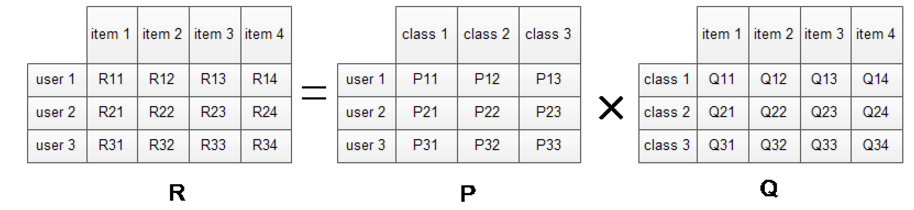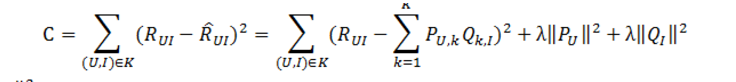LSA模型

Latent Semantic Analysis (Latent Semantic Indexing)

LSA(LSI)使SVD $LSA(LSI)使用SVD$来对单词-文档矩阵进行分解。 SVD $SVD$可以看作是从单词-文档矩阵中发现不相关的索引变量(因子)，将原来的数据映射到语义空间内。在单词-文档矩阵中不相似的两个文档，可能在语义空间内比较相似。

SVD $SVD$，亦即奇异值分解 ，一个 td $t*d$维的(单词-文档矩阵) X $X$，可以分解为:

X=TSDT

X=TSDT

Dirichlet分布+多项式分布=Dirichlet分布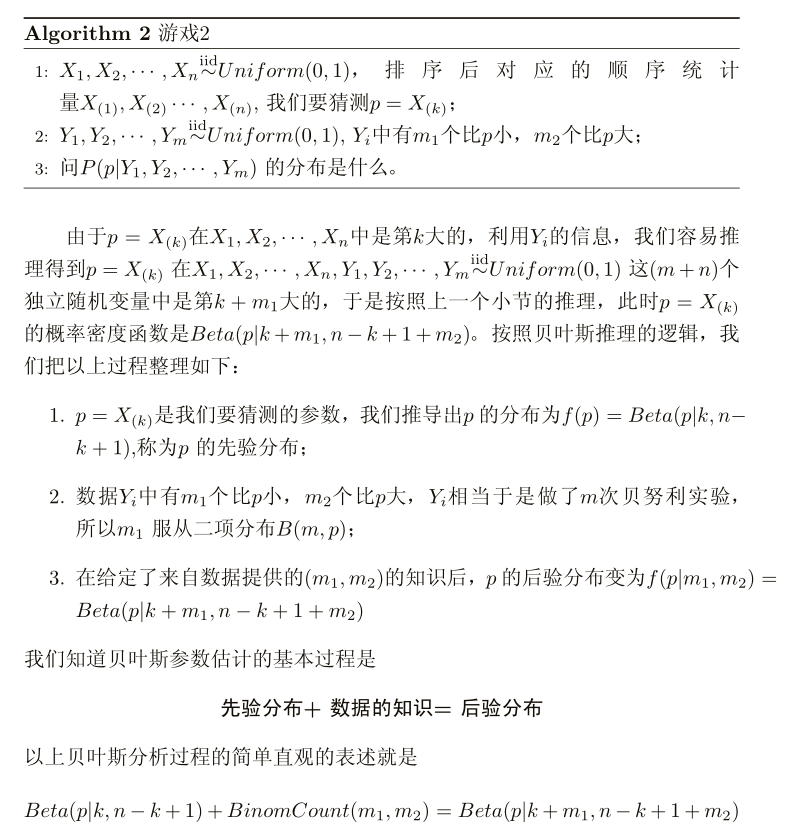PLSA模型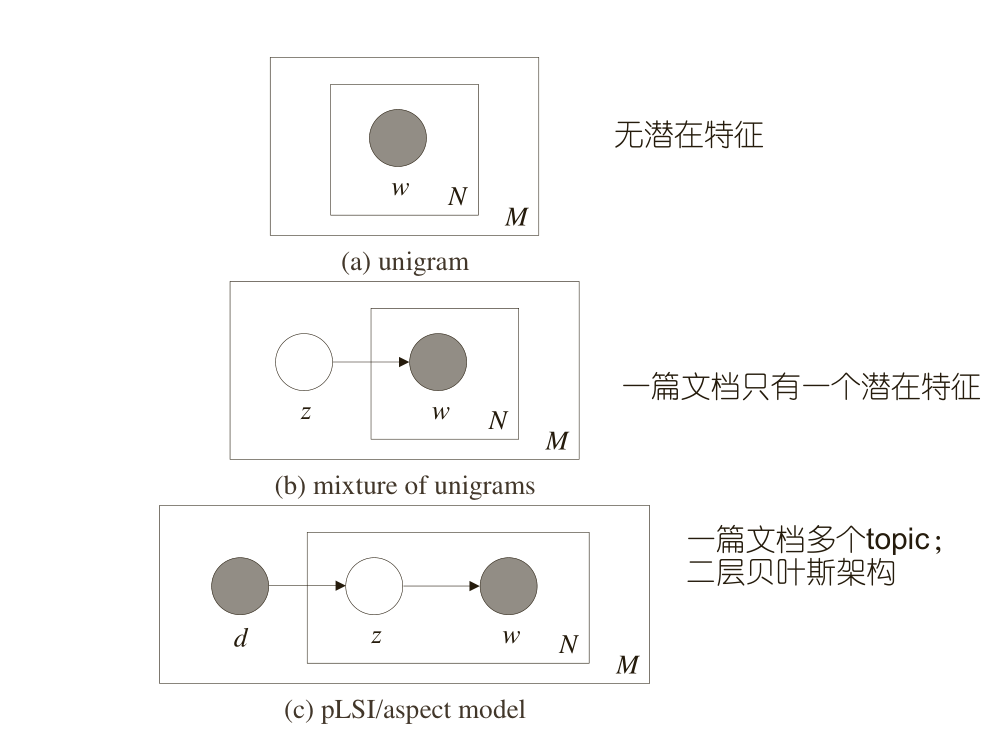p(W)=dDiNp(wi)=dDvVp(wv)cv

p(W,z|d)=p(z|d)iNp(wi|z)p(W|d)=zp(z|d)iNp(wi|z)

p(W,θ)=p(θ)p(W|θ)p(W)=p(θ)p(W|θ)dθ=p(θ)p(wi|θ)dθ

p(wi|dm)=zp(wi|z)p(z|dm)p(W|dm)=iNzp(wi|z)p(z|dm)=iNzθwi,zϕdm

LDA模型

PLSA 模型本质上是频率学派思想，我们现在利用贝叶斯思想进行考虑；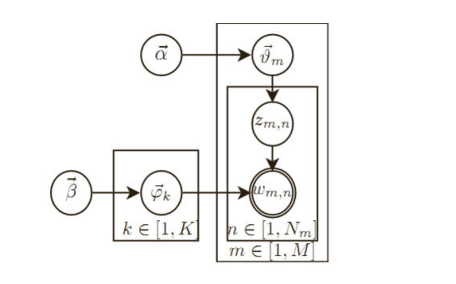p(Z|α)=mMp(zm|θm)p(θm|α)dθm=mMnNp(θm,n)nznDir(θm|α)dθm=mMnNp(θm,n)nzn1(α)nNp(θm,n)α1dθm=mM1(α)nNp(θm,n)nn+α1dθm=mM(nm+α)(α)

p(W|Z,β)=kKp(W(k)|Z(k),β)=kK(nk+β)(β)

p(W,Z|α,β)=p(Z|α)p(W|Z,β)=kK(nk+β)(β)mM(nm+α)(α)

（注：这里可以使用变分EM模型解耦，然后估计隐变量Z的分布；另一种是使用gibbs 采样进行估计）；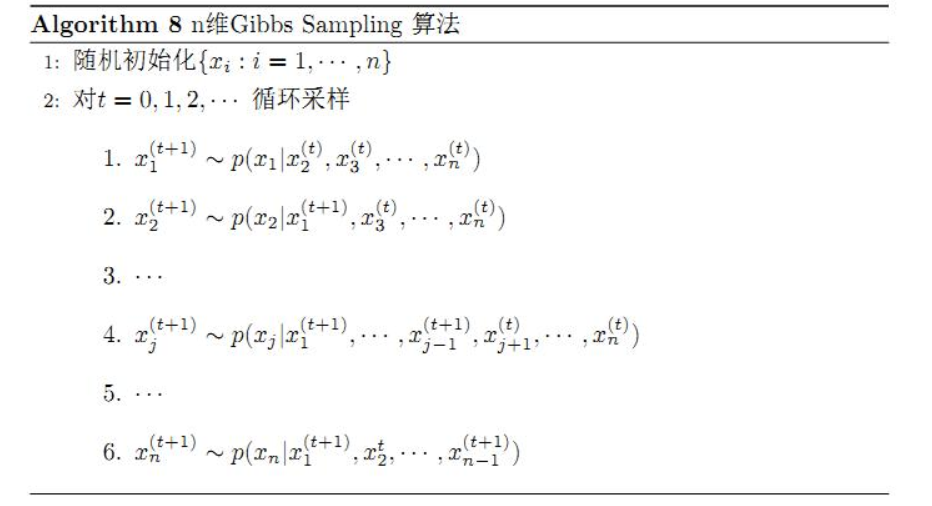gibbs 采样需要已知条件概率，所以我们继续推导如下：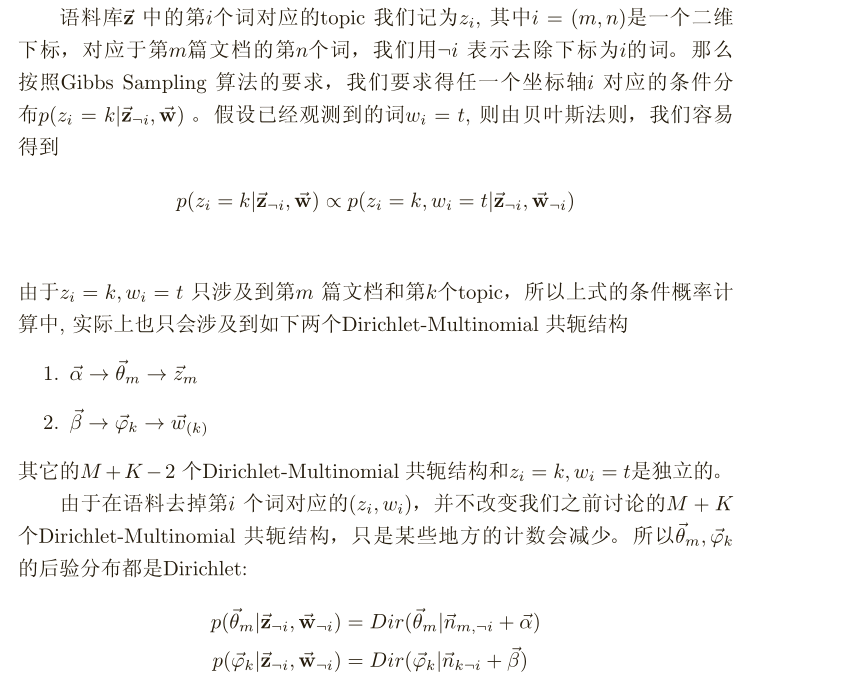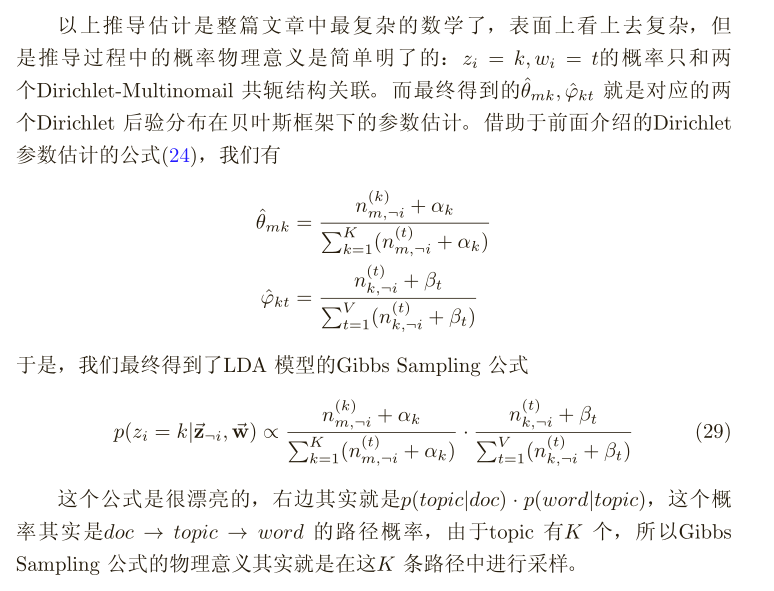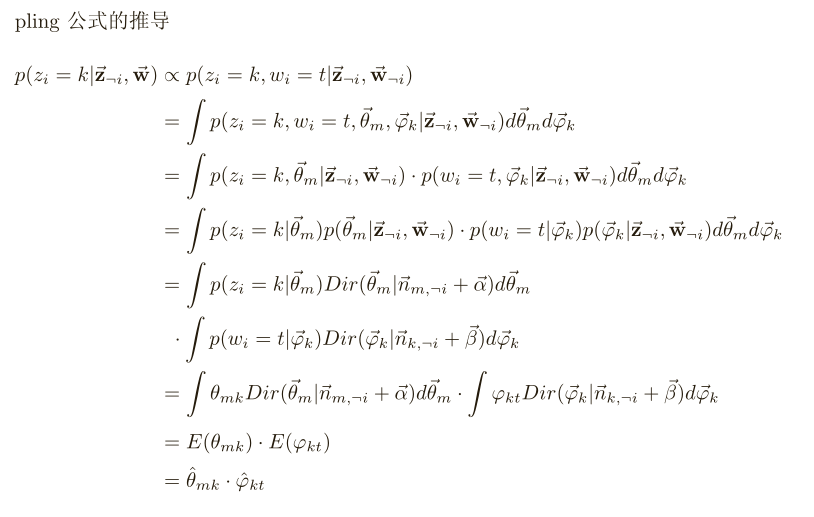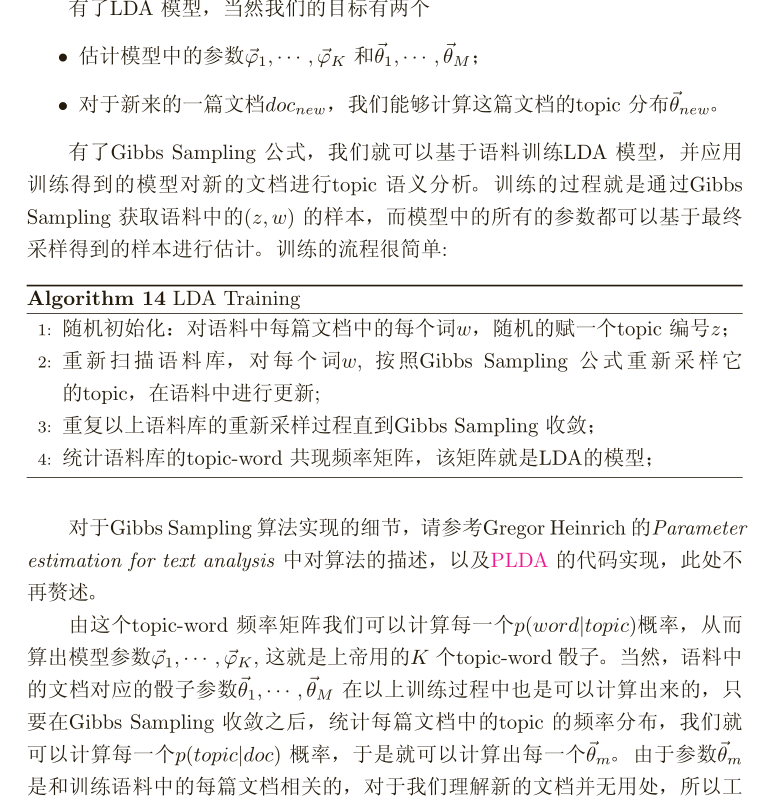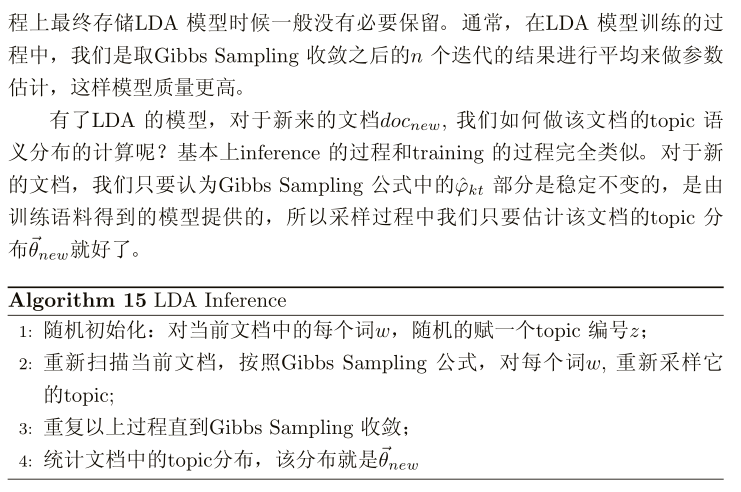11-18721
05-124712-19168
03-12172
08-021万+
04-198607
12-164万+
09-3027
08-272318
01-124万+
09-24801
12-31205
02-165260
07-137961
05-16639
09-211万+
01-10104
03-08431
05-232225
04-122580¥2 ¥4 ¥6 ¥10 ¥20余额支付 (余额：-- )扫码支付获取中扫码支付点击重新获取扫码支付1.余额是钱包充值的虚拟货币，按照1:1的比例进行支付金额的抵扣。
2.余额无法直接购买下载，可以购买VIP、C币套餐、付费专栏及课程。余额充值